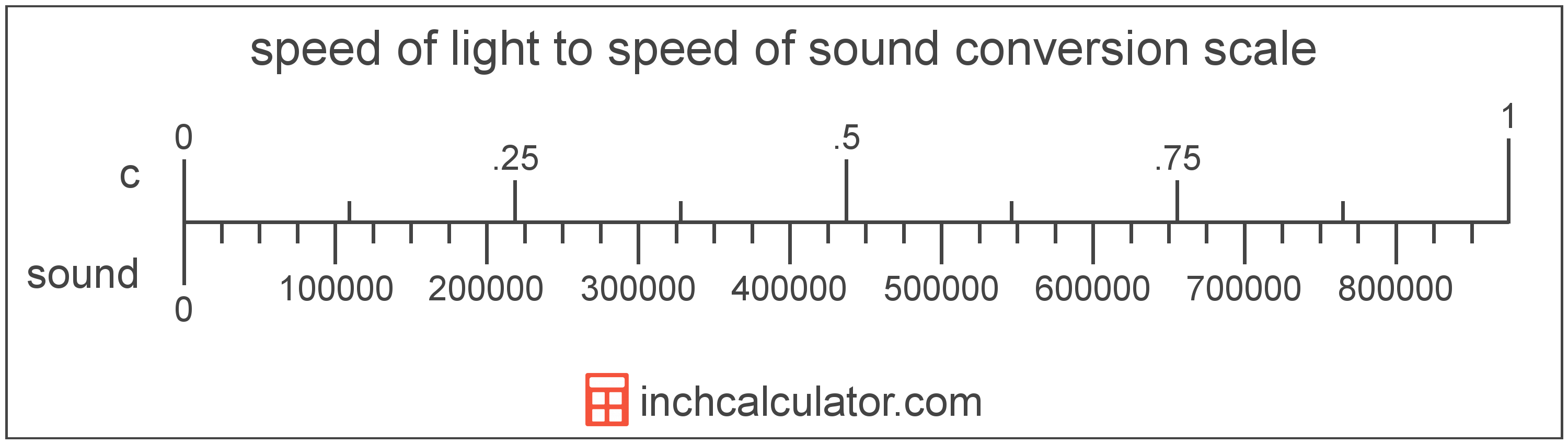# Speed of Light to Speed of Sound Converter

Enter the speed in speed of light below to get the value converted to speed of sound.

Results in Speed of Sound:1 c = 874,030.489796 sound

Do you want to convert speed of sound to speed of light?

## How to Convert Speed of Light to Speed of Sound

To convert a measurement in speed of light to a measurement in speed of sound, multiply the speed by the following conversion ratio: 874,030.489796 speed of sound/speed of light.

Since one speed of light is equal to 874,030.489796 speed of sound, you can use this simple formula to convert:

speed of sound = speed of light × 874,030.489796

The speed in speed of sound is equal to the speed in speed of light multiplied by 874,030.489796.

For example, here's how to convert 5 speed of light to speed of sound using the formula above.
speed of sound = (5 c × 874,030.489796) = 4,370,152.44898 soundSpeed of light and speed of sound are both units used to measure speed. Keep reading to learn more about each unit of measure.

## What is the Speed of Light?

The speed of light is equal to exactly 299,792,458 meters per second, or 670,616,629 miles per hour. The definition of the speed of light is actually derived from the most recent 1983 international definition of the meter.

Speed of light can be abbreviated as c; for example, 1 speed of light can be written as 1 c.

## What is the Speed of Sound?

The speed of sound is the distance a sound wave travels through an elastic medium. The speed of sound through air at 20 °C is equal to 343 meters per second, or roughly 767 miles per hour.

Speed of sound can be abbreviated as sound; for example, 1 speed of sound can be written as 1 sound.

## Speed of Light to Speed of Sound Conversion Table

Table showing various speed of light measurements converted to speed of sound.
Speed Of Light Speed Of Sound
0.00001 c 8.7403 sound
0.00002 c 17.48 sound
0.00003 c 26.22 sound
0.00004 c 34.96 sound
0.00005 c 43.7 sound
0.00006 c 52.44 sound
0.00007 c 61.18 sound
0.00008 c 69.92 sound
0.00009 c 78.66 sound
0.000001 c 0.87403 sound
0.00001 c 8.7403 sound
0.0001 c 87.4 sound
0.001 c 874.03 sound
0.01 c 8,740 sound
0.1 c 87,403 sound
1 c 874,030 sound

## References

1. National Institute of Standards and Technology, SI Redefinition: Meter, https://www.nist.gov/si-redefinition/meter
2. CK-12, Speed of Sound, https://www.ck12.org/physics/speed-of-sound/lesson/Speed-of-Sound-MS-PS/